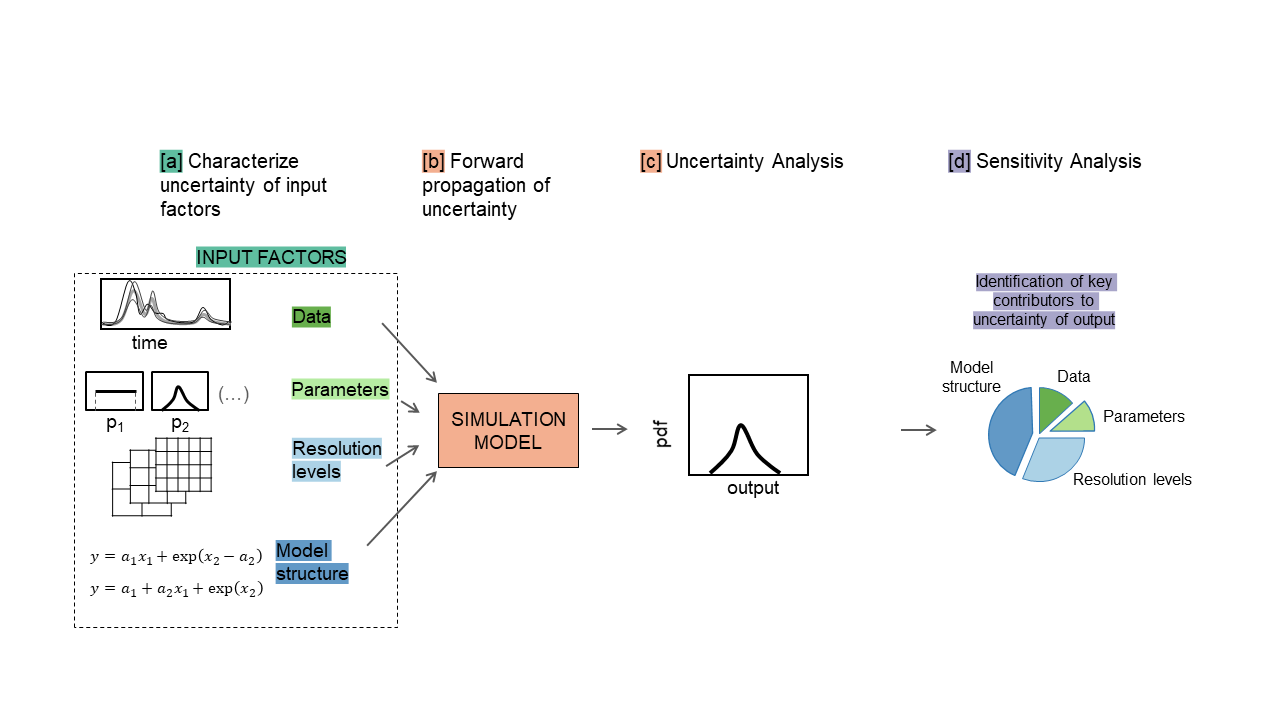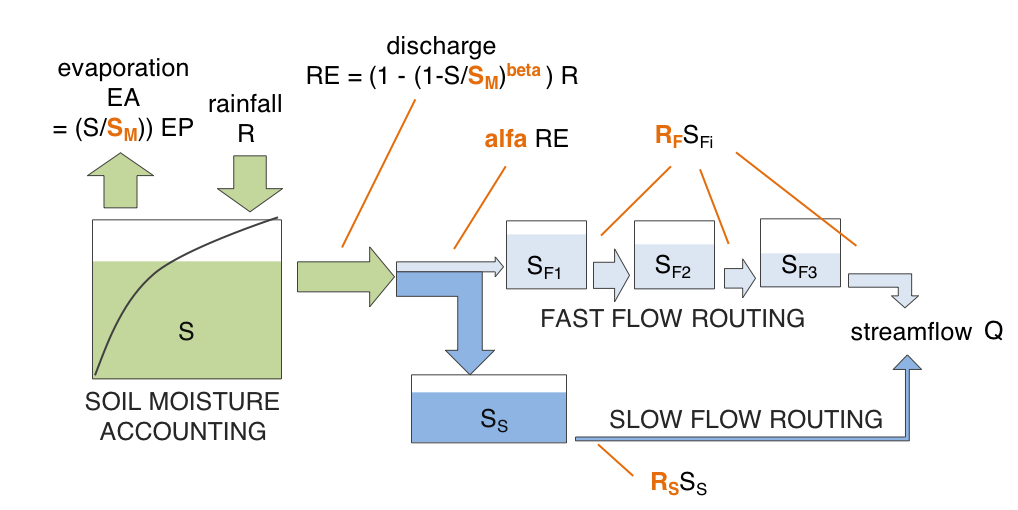# Interactive Jupyter Notebooks for the visual analysis of critical choices in Global Sensitivity Analysis¶

### (Department of Civil Engineering, University of Bristol, UK)¶

This document provides:

• a brief introduction to Global Sensitivity Analysis (GSA);
• a workflow to asssess one of the critical choices needed to set up a GSA application. Here we use the SAFE (Sensitivity Analysis For Everybody) toolbox (References 1-2).

The critical choice that will be considered is:

The impact on the GSA results of the definition of the space of variability of the input factors.

In this example we apply the Regional Sensitivity Analysis GSA method to the rainfall-runoff Hymod model.

# PART I: Introduction¶

## What is Global Sensitivity Analysis and why shall we use it?¶

Global Sensitivity Analysis is a set of mathematical techniques which investigate how the uncertainty in the model inputs influences the variability of the model outputs (References 3-4).

The benefits of applying GSA are:

• Better understanding of the model: Evaluate the model behaviour beyond default set-up

• “Sanity check” of the model: Test whether the model "behaves well" (model validation)

• Priorities for uncertainty reduction: Identify the important inputs on which to focus computationally-intensive calibration, acquisition of new data, etc.

• More transparent and robust decisions: Understand how assumptions about uncertain inputs reflect on the modelling outcome and thus on model-informed decisions

## How Global Sensitivity Analysis works¶

GSA investigates how the uncertainty in the model input factors influences the variability of the model output.

An 'input factor' is any element that can be changed before running the model. In general, input factors could be the model's parameters, forcing input data, but also the very equations implemented in the model or other set-up choices (for instance, the spatial resolution) needed for the model execution on a computer.

An 'output' is any variable that is obtained after the model execution.

The main steps to perform GSA are summarised in the figure below.a. The input factors are sampled from their ranges of variability.

b. The model is repeatedly run against each of the input sampled combinations.

c. The output samples so obtained can be used to characterise the output uncertainty, for instance through a probability distribution or scatter plots.

d. GSA is applied to the input and output samples in order to obtain a set of sensitivity indices. The sensitivity indices measure the relative influence of each input factor on the uncertainty of the output (References 4,5).

# PART II: Workflow application¶

### Step 1: Import python modules¶

In :
from __future__ import division, absolute_import, print_function

import numpy as np
import pandas as pd
import matplotlib as mpl
import matplotlib.pyplot as plt
import scipy.stats as st
import pandas as pd
import plotly.express as px
from plotly.subplots import make_subplots
import plotly.graph_objects as go
from ipywidgets import widgets

# Import SAFE modules:
import os
mydir = "C:/Users/valen/OneDrive - University of Bristol/proj_SAFEVAL/SAFEPy/SAFEpython_v0.0.0"
os.chdir(mydir + "/SAFEpython")

import SAFEpython.RSA_groups as Rg # module to perform RSA with groups
import SAFEpython.plot_functions as pf # module to visualize the results
from SAFEpython.model_execution import model_execution # module to execute the model
from SAFEpython.sampling import AAT_sampling, AAT_sampling_extend # module to perform the input sampling
from SAFEpython.util import aggregate_boot  # function to aggregate the bootstrap results
from SAFEpython import HyMod


### Test model: Rainfall-runoff Hymod model¶

Before applying GSA, let's have a brief overview of the HyMod model, which it is a parsimonious rainfall-runoff model based on the theory of runoff yeild under infiltration excess.

Hymod (Boyle 2001; Wagener et al. 2001) is composed of a soil moisture accounting routine, and a flow routing routine, which in its turn is composed of a fast and a slow routing pathway.

The model structure is illustrated in the figure below.### Step 2: Setup the model¶

Define:

• the input factors whose influence is to be analysed with GSA,
• their range of variability (choice made by expert judgement, available data or previous studies),
• choice of their distributions.

Define input factors of interest:

In :
M = 5 # number of input factors
col_names = ["$S_M$" , "$beta$" , "$alpha$", "$R_S$", "$R_F$"] # input factors of interest


Define their range of variability:

In :
data = [["mm"  , 1   , 400 , "Maximum storage capacity"],
["-"   , 0   , 2   , "Degree of spatial variability of the $S_M$"],
["-"   , 0, 1, "Factor distributing slow and quick flows"],
["1/dd", 0, 0.1, "Fractional discharge of the slow release reservoir"],
["1/dd", 0.1, 1, "Fractional discharge of the quick release reservoirs"]]
model_inputs = pd.DataFrame(data,
columns=["Unit", "Min value", "Max value", "Description"],
index = ["$S_M$" , "$beta$" , "$alpha$", "$R_S$", "$R_F$"])
model_inputs

Out:
Unit Min value Max value Description
$S_M$ mm 1.0 400.0 Maximum storage capacity
$beta$ - 0.0 2.0 Degree of spatial variability of the $S_M$
$alpha$ - 0.0 1.0 Factor distributing slow and quick flows
$R_S$ 1/dd 0.0 0.1 Fractional discharge of the slow release reser...
$R_F$ 1/dd 0.1 1.0 Fractional discharge of the quick release rese...

Define choice of their distributions:

In :
distr_fun = st.uniform # uniform distribution
distr_par = [np.nan] * M
samp_strat = 'lhs' # Latin Hypercube
fun_test = HyMod.hymod_MulObj
N = 2000 # number of samples

In :
# Load data:
data = np.genfromtxt(mydir +'/data/LeafCatch.txt', comments='%')
rain = data[0:365, 0] # 1-year simulation
evap = data[0:365, 1]
flow = data[0:365, 2]
warmup = 30 # Model warmup period (days)

In :
class setup_model:
def __init__(self, x1, x2, x3, x4, x5):
# The shape parameters of the uniform distribution are the lower limit and the difference between lower and upper limits:
self.xmin = [x1.value, x2.value, x3.value, x4.value, x5.value]
self.xmax = [x1.value, x2.value, x3.value, x4.value, x5.value]
for i in range(M):
distr_par[i] = [self.xmin[i], self.xmax[i] - self.xmin[i]]
self.distr_par = distr_par


### Step 3: Sample inputs space¶

The number of model runs (N) typically increases proportionally to the number of input factors ($M$) and will depend on the GSA methods chosen as well.

In :
class sample_input:
def __init__(self,distr_par):
self.X = AAT_sampling(samp_strat, M, distr_fun, distr_par, N)


### Step 4: Run the model¶

For each sampled input factors combination, we run the model and save the associated model output.

In :
class run_model:
def __init__(self,X):
self.Y = model_execution(fun_test, X, rain, evap, flow, warmup)


### Step 5: Apply Sensitivity Analysis (RSA) method¶

This implementation of the RSA method for Sensitivity Analysis requires to sort the output samples and then to split them into a certain number of groups (defined by the user). Afterwards, RSA identifies the sub-samples in the inputs space that produced the outputs in each group and compute the cumulative distribution function (CDF) of each sub-sample. Finally, the sensitivity indices are defined as the (median of the) maximum vertical distance between the CDFs of the various groups.

Here we divide the output samples into 10 groups, where each group contains the same number of samples.

In :
class RSA_method:
def __init__(self,X,Y,Nboot):
mvd_median, mvd_mean, mvd_max, spread_median, spread_mean, spread_max, self.idx, self.Yk = \
Rg.RSA_indices_groups(X, Y, ngroup=10, Nboot=Nboot.value)

self.mvd_p = pd.DataFrame(mvd_median,columns=model_inputs.index)
Xdf = pd.DataFrame(X,columns=[col_names])
xx = pd.melt(Xdf)
Ydf = pd.DataFrame(Y,columns=["y"])
yy = pd.concat([Ydf]*M,ignore_index=True)
self.new_data = pd.concat([xx,yy],axis=1)
self.new_data.columns = ["Input","x","y"]
all_ind = np.concatenate((self.idx,self.idx,self.idx,self.idx,self.idx),axis=0)
all_ind = pd.DataFrame(all_ind,columns=['idx'])
self.new_data['idx'] = all_ind


### Step 6: Check model behaviour by visualising input/output samples¶

Scatterplots are plotted to visualise the behaviour of the output over each input factor in turn.

Definition of interactivity

In :
def update_figures(change):
with fig.batch_update():
distr_par = setup_model(x1, x2, x3, x4, x5).distr_par
X = sample_input(distr_par).X
Y = run_model(X).Y[:,0]
RSA = RSA_method(X,Y,Nboot)
new_data = RSA.new_data
fig.data.x = new_data.loc[new_data['Input'] == model_inputs.index, 'x']
fig.data.x = new_data.loc[new_data['Input'] == model_inputs.index, 'x']
fig.data.x = new_data.loc[new_data['Input'] == model_inputs.index, 'x']
fig.data.x = new_data.loc[new_data['Input'] == model_inputs.index, 'x']
fig.data.x = new_data.loc[new_data['Input'] == model_inputs.index, 'x']
fig.data.marker.color = new_data.loc[new_data['Input'] == model_inputs.index, 'idx']
fig.data.marker.color = new_data.loc[new_data['Input'] == model_inputs.index, 'idx']
fig.data.marker.color = new_data.loc[new_data['Input'] == model_inputs.index, 'idx']
fig.data.marker.color = new_data.loc[new_data['Input'] == model_inputs.index, 'idx']
fig.data.marker.color = new_data.loc[new_data['Input'] == model_inputs.index, 'idx']
fig.data.y = new_data.loc[new_data['Input'] == model_inputs.index, 'y']
fig.data.y = new_data.loc[new_data['Input'] == model_inputs.index, 'y']
fig.data.y = new_data.loc[new_data['Input'] == model_inputs.index, 'y']
fig.data.y = new_data.loc[new_data['Input'] == model_inputs.index, 'y']
fig.data.y = new_data.loc[new_data['Input'] == model_inputs.index, 'y']
fig.layout.barmode = 'overlay'

mvd_p = RSA.mvd_p
for i in range(0,5):
fig1.data[i].y = np.array(mvd_p[model_inputs.index[i]])


Definition of the sliders

In :
x1 = widgets.FloatRangeSlider(value = [0, 400], min = 0, max = 800, step = 1, description = model_inputs.index,
readout_format = '.1f', continuous_update=False)
x1.observe(update_figures,names = 'value')

x2 = widgets.FloatRangeSlider(value = [0, 2], min = 0, max = 4, step = 0.1, description = model_inputs.index,
readout_format = '.1f', continuous_update=False)
x2.observe(update_figures,names = 'value')

x3 = widgets.FloatRangeSlider(value = [0, 1], min = 0, max = 1, step = 0.1, description = model_inputs.index,
readout_format = '.1f', continuous_update=False)
x3.observe(update_figures,names = 'value')

x4 = widgets.FloatRangeSlider(value = [0, 0.1], min = 0, max = 0.4, step = 0.01, description = model_inputs.index,
readout_format = '.1f', continuous_update=False)
x4.observe(update_figures,names = 'value')

x5 = widgets.FloatRangeSlider(value = [0.1, 1], min = 0.1, max = 1, step = 0.1, description = model_inputs.index,
readout_format = '.1f', continuous_update=False)
x5.observe(update_figures,names = 'value')

Nboot = widgets.IntSlider(value = 2, min = 2, max = 200, step = 1, description = "Nboot",
continuous_update=False)
Nboot.observe(update_figures,names = 'value')


Definition of the figure

In :
distr_par = setup_model(x1, x2, x3, x4, x5).distr_par
X = sample_input(distr_par).X
Y = run_model(X).Y[:,0]
RSA = RSA_method(X,Y,Nboot)
new_data = RSA.new_data
p = px.scatter(new_data, x = "x", y = 'y', facet_col = "Input",color="idx",
color_continuous_scale = px.colors.diverging.RdYlBu[::-1],
width=1000, height=500)
fig = go.FigureWidget(data=p, layout=go.Layout(barmode='overlay'))
fig.update_xaxes(title_text = "")
fig.update_xaxes(matches=None)
fig.layout.yaxis.title="RMSE of streamflow prediction"
fig.show()